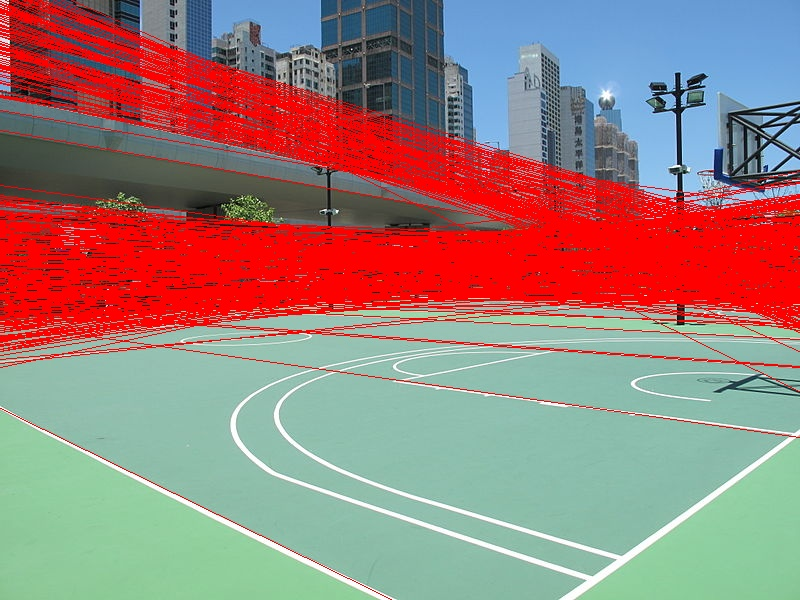# Dec 02 2016 Detecting lines in an image with Hough transforms

Part of a series of examples from the CV cheat sheet. Click here for other computer vision applications.

Detecting straight lines in an image is a common task in computer vision. One way to do this is with the Hough (pronounced "Huff") transform. The math behind it is beyond the scope of this example, but we should be able to get you off the ground using it with OpenCV and Python. We're going to assume you have OpenCV installed already and are able to use it in Python.

Take an input image, say, this basketball court:Credit: Wikimedia Commons

Let's detect the straight lines in this image. The gist is this:

1. Open an image
2. Detect edges in it
3. Use those edges to detect straight lines
4. Draw those lines back on the image (you don't need to do this necessarily, but it's a nice visual)

Here's the code to extract lines in Python:

 ``` 1 2 3 4 5 6 7 8 9 10 11 12 13 14 15 16 17 18 19 20 21 22 23 24 25 26 27 28 29 30 31 32 33 34 35 36 37 38``` ``````# import libraries we'll need import cv2 import numpy as np # read in your image img = cv2.imread('court.jpg') # convert to grayscale so we can detect edges gray = cv2.cvtColor(img, cv2.COLOR_BGR2GRAY) # use Canny edge detector to find edges in the image. The thresholds determine how # weak or strong an edge will be detected. These can be tweaked. lower_threshold = 50 upper_threshold = 150 edges = cv2.Canny(gray, lower_threshold, upper_threshold) # detect lines in the image. This is where the real work is done. Higher threshold # means a line needs to be stronger to be detected, so again, this can be tweaked. threshold = 250 lines = cv2.HoughLines(edges, 1, np.pi / 180, threshold) # convert each line to coordinates back in the original image for line in lines: for rho, theta in line: a = np.cos(theta) b = np.sin(theta) x0 = a * rho y0 = b * rho x1 = int(x0 + 1000 * -b) y1 = int(y0 + 1000 * a) x2 = int(x0 - 1000 * -b) y2 = int(y0 - 1000 * a) # draw each line on the image cv2.line(img, (x1, y1), (x2, y2), (0, 0, 255), 1) # write the image to disk cv2.imwrite('houghlines.jpg', img) ``````

Here's the result:We can see it found the majority of lines on the court - and quite a few in the skyline. This is why you'll want to tune the threshold in `cv2.HoughLines(edges, 1, np.pi / 180, threshold)` based on your needs to prevent false positives or negatives.